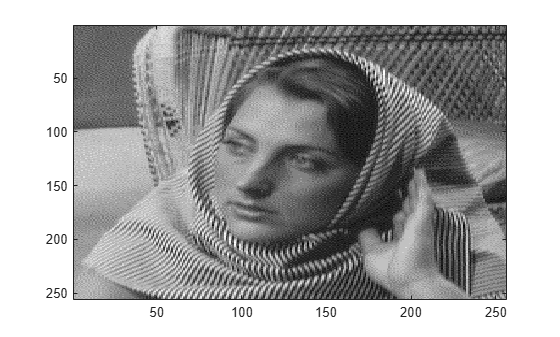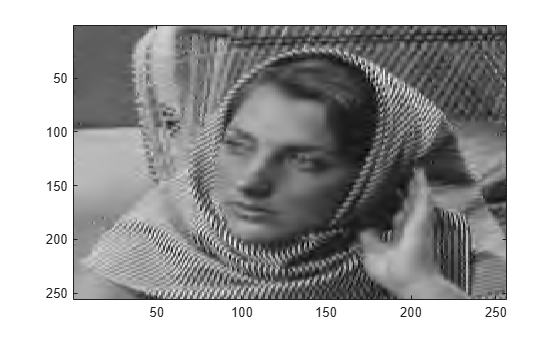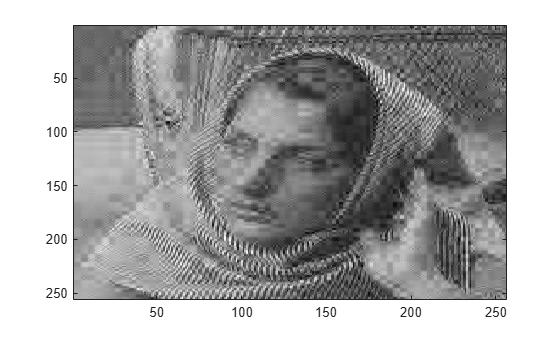Image Reconstruction with Biorthogonal Wavelets

This example shows how applying the order biorthogonal wavelet filters can affect image reconstruction.

Generate the analysis and synthesis filters for the bior3.5 wavelet. Load in and display an image.

[LoD,HiD,LoR,HiR] = wfilters('bior3.5');
imagesc(X)
colormap grayThe analysis filters, LoD and HiD, have 5 vanishing moments. The synthesis filters, LoR and HiR, have 3 vanishing moments. Do a five-level wavelet decomposition of the image using the analysis filters.

[c1,s1] = wavedec2(X,5,LoD,HiD);

Find the threshold that keeps only those wavelet coefficients with magnitudes in the top 10 percent. Use the threshold to set the bottom 90 percent of coefficients to 0.

frac = 0.1;
c1sort = sort(abs(c1),'desc');
num = numel(c1);
thr = c1sort(floor(num*frac));
c1new = c1.*(abs(c1)>=thr);

Reconstruct the image using the synthesis filters and the thresholded coefficients. Display the reconstruction.

X1 = waverec2(c1new,s1,LoR,HiR);
figure
imagesc(X1)
colormap grayDo a five-level wavelet decomposition of the image using the synthesis filters.

[c2,s2] = wavedec2(X,5,LoR,HiR);

Find the threshold that keeps only those wavelet coefficients with magnitudes in the top 10 percent. Use the threshold to set the bottom 90 percent of coefficients to 0

frac = 0.1;
c2sort = sort(abs(c2),'desc');
num = numel(c2sort);
thr = c2sort(floor(num*frac));
c2new = c2.*(abs(c2)>=thr);

Reconstruct the image using the synthesis filters and the thresholded coefficients. Display the reconstruction. Decomposing with a filter that has 3 vanishing moments and reconstructing with a filter that has 5 vanishing moments results in poor reconstruction.

X2 = waverec2(c2new,s2,LoD,HiD);
figure
imagesc(X2)
colormap gray